Next: Mutual information and Kullback-Leibler Up: Multi-unit contrast functions Previous: Multi-unit contrast functions

### Likelihood and network entropy

It is possible to formulate the likelihood in the noise-free ICA model (11), which was done in , and then estimate the model by a maximum likelihood method. Denoting bythe matrix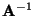, the log-likelihood takes the form :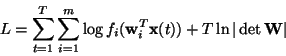(13)

where the fi are the density functions of the si (here assumed to be known), and the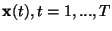are the realizations of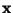.

Another related contrast function was derived from a neural network viewpoint in [12,108]. This was based on maximizing the output entropy (or information flow) of a neural network with non-linear outputs. Assume thatis the input to the neural network whose outputs are of the form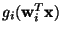, where the gi are some non-linear scalar functions, and the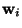are the weight vectors of the neurons. One then wants to maximize the entropy of the outputs: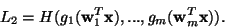(14)

If the gi are well chosen, this framework also enables the estimation of the ICA model. Indeed, several authors, e.g., [23,123], proved the surprising result that the principle of network entropy maximization, or 'infomax', is equivalent to maximum likelihood estimation. This equivalence requires that the non-linearities gi used in the neural network are chosen as the cumulative distribution functions corresponding to the densities fi, i.e., gi'(.)=fi(.).

The advantage of the maximum likelihood approach is that under some regularity conditions, it is asymptotically efficient; this is a well-known result in estimation theory . However, there are also some drawbacks. First, this approach requires the knowledge of the probability densities of the independent components. These could also be estimated [124,96], but this complicates the method considerably. A second drawback is that the maximum likelihood solution may be very sensitive to outliers, if the pdf's of the independent components have certain shapes (see ), while robustness against outliers is an important property of any estimator [50,56]4.Next: Mutual information and Kullback-Leibler Up: Multi-unit contrast functions Previous: Multi-unit contrast functions
Aapo Hyvarinen
1999-04-23